Random element

Subsequently, the term "random element" was used chiefly with reference to "randomly chosen" element in some linear topological space, especially a Hilbert space or a Banach space. The exact definition of a random elementin a Banach space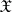, for example, is reminiscent of the definition of a random variable. Let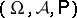be some probability space, letbe a Banach space and let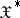be the dual space of. A mapping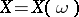from the spaceof elementary eventsintois called a random element if every continuous linear functional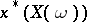is actually a random variable, that is, an-measurable function.
Let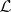be the smallest-algebra of subsets ofwith respect to which all continuous linear functionals are measurable.is a random element if and only if the complete pre-image of all sets inare-measurable. In the case whenis separable,coincides with the-algebra of Borel subsets of.
The basic concepts of probability theory, such as the characteristic function, the mathematical expectation and the covariance, among other things, can be extended to random elements. A random elementis called normal (Gaussian) if the probability distribution of every continuous linear functional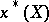is normal (cf. Normal distribution). The weak law of large numbers, the strong law of large numbers, the law of the iterated logarithm, the central limit theorem, and other assertions of probability theory can be extended to sequences of random elements. Whether these theorems in their classical form carry over to the case of Banach spaces depends on the geometry of the space. It is important to note that this is a two-way connection, in that the probabilistic properties often turn out to be in fact probabilistic-geometric: not only is their validity in a given Banach space determined by the geometric properties of the space, but conversely it determines these properties. E.g., for any sequence of independent identically-distributed random elements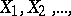with values in, zero mathematical expectations and, the distribution of the normalized sum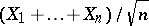converges weakly to the distribution of a normal random element asif and only ifis a so-called type-2 space (see ).